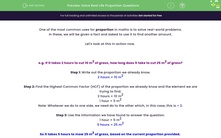# Solve Real Life Proportion Questions

In this worksheet, students will solve real life proportion problems with two or more variables using the methods of applying and manipulating the information provided in the question.Key stage:  KS 4

Year:  GCSE

GCSE Subjects:   Maths

GCSE Boards:   AQA, Eduqas, Pearson Edexcel, OCR,

Curriculum topic:   Ratio, Proportion and Rates of Change

Curriculum subtopic:   Ratio, Proportion and Rates of Change Direct and Inverse Proportion

Difficulty level:#### Worksheet Overview

One of the most common uses for proportion in maths is to solve real-world problems.

In these, we will be given a fact and asked to use it to find another amount.

Let's look at this in action now.

e.g. If it takes 2 hours to cut 10 m2 of grass, how long does it take to cut 25 m2 of grass?

Step 1: Write out the proportion we already know.

2 hours = 10 m2

Step 2: Find the Highest Common Factor (HCF) of the proportion we already know and the element we are trying to find.

2 hours = 10 m2

1 hour = 5 m2

Note: Whatever we do to one side, we need do to the other which, in this case, this is ÷ 2.

Step 3: Use the information we have found to answer the question.

1 hour = 5 m2

5 hours = 25 m2

So it takes 5 hours to mow 25 m2 of grass, based on the current proportion provided.

e.g. 4 people take 3 hours to clean 60 cars. How long will it take 2 people to clean 80 cars?

This one is a little more complicated as we have three variables present.

Our first step is to write out what we know as an equation:

4 people = 3 hours = 60 cars

The key step to apply here is that we can only change two of the three quantities at once.

We need to keep testing and making changes to two elements at a time, until we have the cars as 80 and the people as 2.

Change 1:

4 people = 3 hours = 60 cars    ÷ Both by 3

So if the same amount of people are cleaning one-third of the cars, it will take one-third of the time:

4 people = 1 hour = 20 cars

Change 2:

4 people = 1 hour = 20 cars    × Both by 4

So if the same amount of people are cleaning 4 times as many cars, it will take them 4 times as long:

4 people = 4 hours = 80 cars

Change 3:

4 people = 4 hours = 80 cars    ÷ People by 2, doubles the hours required

So if half as many people are cleaning the same amount of cars, it will take twice as long:

2 people = 8 hours = 80 cars

So based on the proportion given, it will take 2 people 8 hours to clean 80 cars.

In this activity, we will solve real life proportion problems with two or more variables using the methods shown above of applying and manipulating the information provided in the question.

### What is EdPlace?

We're your National Curriculum aligned online education content provider helping each child succeed in English, maths and science from year 1 to GCSE. With an EdPlace account you’ll be able to track and measure progress, helping each child achieve their best. We build confidence and attainment by personalising each child’s learning at a level that suits them.

Get started